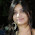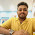Inside Data Structures and Algorithms

I'm a sophomore now and have Data Structures and Algorithms in this semester. They're the most important stuff to learn in computer science and programming without being strong in it, one will just remain a monkey coder.

In Computer Science, from processor to RAM, operating system to cloud database, everywhere we work with data. We manipulate them, process them and then yield desired results. Handling data efficiently and precisely is a very crucial task. Ever since the computing era has begun, human minds have shown eagerness to develop better and efficient techniques of working with data which is only because to reduce time consumption in yielding results and also to save storage space.

First of all, what is data?

Data is any set of values. It can be the depth of river Ganga or the marksheet of your last semester. In computing, data is all about binary digits $$0s$$ and $$1s$$ (bits or binary digits are the smallest unit of data). Computers are basically electronic machines and is constructed of billions of ON/OFF switches (transistors). 1 is the ON state of a transistor and 0 is the OFF. A computer uses binary digits to store all of our real world data. Data is collected, measured, recorded and organized.

How can we organize data?

Imagine there is an office where files are scattered everywhere, and another office where files are neatly placed one above the other. Now, if you were supposed to find a particular file then which office's arrangement would make your task easier? Obviously, the latter one. Why? Because it is much easier to find a particular file from an organized stack of files. Here, intuitively the concept of data structures has been used.

What is Data Structure?

Data structure is a way of storing and organizing data to access and use data efficiently.
There are two types of data structures on the basis of organization of the elements:-
• Linear data structure - Elements are accessed in a sequential order but it is not compulsory to store all elements sequentially. It can only have one successor and one predecessor. Examples: arrays, linked lists, stacks and queues.
• Non-linear data structure - Elements of this data structure are stored/accessed in a non-linear order. It can have more than one successor and predecessor. Examples: trees and graphs.
I will discuss each of these stuff in further blog posts, stay tuned.

Can you answer this? Are data structures implemented on data in primary memory i.e. RAM or in secondary memory i.e. hard disk?

Answer - Data structures are implemented on data in RAM, because it is the device where manipulation and processing of data is carried out. Hard disk stores the processed data.

Imagine that you have collected a chunk of data, now you should organize and process it to yield some results. But, how will you do that? Here, comes algorithms in play.

What is an Algorithm?

Written below are the instructions to turn ON a computer:-
1. Switch ON the main power supply.
2. Is there a UPS?
1. If Yes, then turn it ON.
2. If No, then go to step 3.
3. Click the power button on the cabinet.
What I tried to show is we need to follow steps to do any task or solve any problem.
So, an algorithm is a step-by-step procedure to solve a given problem. Every algorithm works on a set of data supplied to it as input, manipulates it with the concept of data structures and produces the output.
Before there were computers, there were algorithms. But now that there are computers, there are even more algorithms, and algorithms lie at the heart of computing.
- excerpt from book, Introduction to Algorithms, CLRS

How good an algorithm can be?

That basically depends on the running time of an algorithm (time complexity) and the space in memory it requires (space complexity). These two factors conclude efficiency of an algorithm. The less is both the factors, the better is the algorithm.

In today's world, our lives are being shaped by algorithms. Algorithms are behind of everything.

References: http://www.bbc.co.uk/education/guides/zwsbwmn/revision

1.Thaanks for this post. Well organized and explained.

1.My pleasure ❤

2.Thanks for this blog bro nd sorry for being late

1.3.4.My pleasure :)